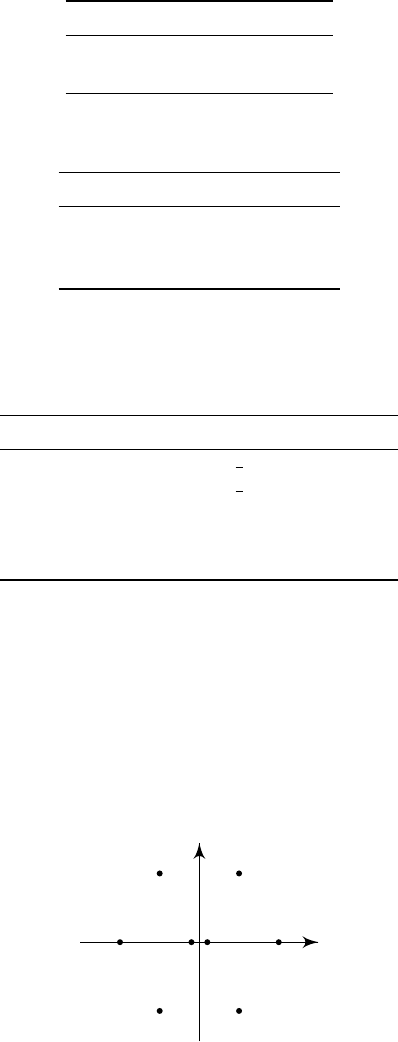8Lie groups in nature

III Symmetries, Fields and Particles8.3 Internal symmetries and the eightfold way
Finally, we get to the notion of internal symmetries. Recall that for a complex
scalar field, our field was invariant under a global U(1) action given by phase
change. More generally, fields can carry some representation of a Lie group
G
.
In general, if this representation has dimension greater than 1, this means that
we have multiple different “particles” which are related under this symmetry
transformation. Then by symmetry, all these particles will have the same mass,
and we get a degeneracy in the mass spectrum.
When we have such degeneracies, we would want to distinguish the different
particles of the same mass. These can be done by looking at the weights of the
representation. It turns out the different weights correspond to the different
quantum numbers of the particles.
Often, we do not have an exact internal symmetry. Instead, we have an
approximate symmetry. This is the case when the dominant terms in the
Lagrangian are invariant under the symmetry, while some of the lesser terms are
not. In particular, the different particles usually have different (but very similar)
masses.
These internal symmetries are famously present in the study of hadrons, i.e.
things made out of quarks. The basic examples we all know are the nucleons,
namely protons and neutrons. We can list them as
Charge (Q) Mass (M)
p +1 938 MeV
n 0 940 MeV
Note that they have very similar masses. Later, we found, amongst many other
things, the pions:
Charge (Q) Mass (M)
π
+
+1 139 MeV
π
0
0 135 MeV
π
-1 139 MeV
Again, these have very similar masses. We might expect that there is some
approximate internal symmetry going on. This would imply that there is some
conserved quantity corresponding to the weights of the representation. Indeed,
we later found one, known as isospin:
Charge (Q) Isospin (J) Mass (M)
p +1 +
1
2
938 MeV
n 0
1
2
940 MeV
π
+
+1 +1 139 MeV
π
0
0 0 135 MeV
π
-1 -1 139 MeV
Isospin comes from an approximate
SU
(2)
I
symmetry, with a generator given by
H = 2J.
The nucleons then have the fundamental ρ
1
representation, and the pions have
the ρ
2
representation.
Eventually, we got smarter, and discovered an extra conserved quantum
number known as hypercharge. We can plot out the values of the isospin and
the hypercharge for our pions and some other particles we discovered, and we
found a pattern:
π
+
K
0
K
+
π
K
¯
K
0
η
π
0
At firsts, physicists were confused by the appearance of this pattern, and tried
very hard to figure out generalizations. Of course, now that we know about Lie
algebras, we know this is the weight diagram of a representation of su(3).
However, the word
SU
(3) hasn’t resolved all the mystery. In reality, we only
observed representations of dimension 1
,
8 and 10. We did not see anything
else. So physicists hypothesized that there are substructures known as quarks.
Each quark (
q
) carry a
3
representation (flavour), and antiquarks (
¯q
) carry a
¯
3
representation.
The mesons correspond to
q¯q
particles, and the representation decompose as
3
¯
3 = 1 8.
Bosons correspond to qqq particles, and these decompose as
3 3 3 = 1 8 8 10.
Of course, we now have to explain why only
q¯q
and
qqq
appear in nature,
and why we don’t see quarks appearing isolated in nature. To do so, we have
to go very deep down in QCD. This theory says that quarks have a
SU
(3)
gauge symmetry (which is a different
SU
(3)) and the quark again carries the
fundamental representation
3
. More details can be found in the Lent Standard
Model course.